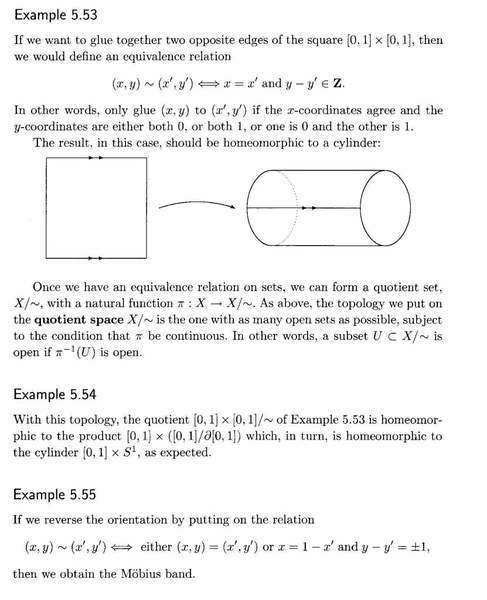# Mobius Band as a Quotient Topology

Gold Member
I am reading Martin Crossley's book, Essential Topology.

I am at present studying Example 5.55 regarding the Mobius Band as a quotient topology.

Example 5.55 Is related to Examples 5.53 and 5.54. So I now present these Examples as follows:I cannot follow the relation $(x,y) \sim (x', y') \Longleftrightarrow \text{ either } (x,y) = (x', y') \text{ or } x = 1 - x' \text{ and } y - y' = \pm 1$

Why do we need $(x,y) = (x', y')$ in the relation? Indeed, why do we need $y - y' = \pm 1$?

Surely all we need is $(x,y) \sim (x', y') \Longleftrightarrow x = 1 - x' \text{ and } y - y' = -1$

Can anyone explain how the relation $(x,y) \sim (x', y') \Longleftrightarrow \text{ either } (x,y) = (x', y') \text{ or } x = 1 - x' \text{ and } y - y' = \pm 1$ actually works to produce the Mobius Band?

Peter

Last edited:

## Answers and Replies

I cannot follow the relation $(x,y) \sim (x', y') \Longleftrightarrow \text{ either } (x,y) = (x', y') \text{ or } x = 1 - x' \text{ and } y - y' = \pm 1$

Why do we need $(x,y) = (x', y')$ in the relation? Indeed, why do we need $y - y' = \pm 1$?

Surely all we need is $(x,y) \sim (x', y') \Longleftrightarrow x = 1 - x' \text{ and } y - y' = -1$

Can anyone explain how the relation $(x,y) \sim (x', y') \Longleftrightarrow \text{ either } (x,y) = (x', y') \text{ or } x = 1 - x' \text{ and } y - y' = \pm 1$ actually works to produce the Mobius Band?

Peter
Well, it's an equivalence relation, so it has to be reflexive by definition. Thus, if ##(x,y)=(x',y')##, then we must have ##(x,y)\sim(x',y')##.

Equivalence relations are symmetric as well, so if ##(x,y)\sim(x',y')##, then we must have ##(x',y')\sim(x,y)##. If ##y-y'=1##, then ##y'-y=-1##.

•1 person